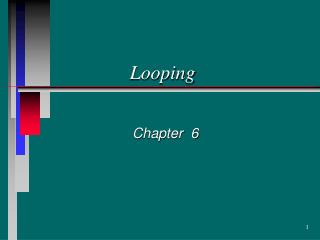DownloadDownload PresentationLooping

# Looping

Télécharger la présentation## Looping

- - - - - - - - - - - - - - - - - - - - - - - - - - - E N D - - - - - - - - - - - - - - - - - - - - - - - - - - -
##### Presentation Transcript

1. Looping Chapter 6

2. Count Controlled Loops Trapping for valid input Using flags to control a while statement Ending a loop with End Of File condition Getting Looped in C++

3. while (condition) false true statement 1 statement 2 The while Statement • Loop <=> a control structure that causes a sequence of statements to be executed repeatedly • Syntax: • The statement can be multiple statements (compound) by using { . . . } while (condition) statement;

4. while (condition) false true statement 1 statement 2 Phases of Loop Execution • Loop entry => flow of control reaches first statement inside loop • Iteration => each pass thru the loop • Loop test => condition tested before each iteration • Loop exit => when termination condition occurs • in while statement, loop is NOT executed another time

5. While Loop Illustration Loop Iteration Loop Test Loop Exit Loop Entry

6. Loops Using the while statement • Count controlled => executes a specified number of times • Event controlled => terminates when something happens inside the loop body to signal that loop should be exited

7. Count Controlled Loop • Uses a Loop Control Variable (LCV) x = 0;while (x <= 10) { cout << “count = “ << x << endl; x = x + 1; } What gets printed?

8. Event Controlled Loops • Sentinel Controlled => look for a special flag or data value What must be known ahead of time?

9. Event Controlled Loops • End of File Controlled Do you need to know how many items are in the file? Why? Why not?

10. Event Controlled Loops • Flag controlled loop How man times will the loop run?

11. Other Uses for Loops • Count how many times something happens

12. 7 Easy Steps for Loop Design • Decide on the termination condition • What is the initial value of the LCV • Where do you update the LCV • What is/are the statement(s) to be repeated • What initialization values must be set • What changes inside the loop (besides the LCV) • What should have happened when loop exits?

13. Amen Count Controlled Loops • Initialized • Incremented • Inspected The LCV must be ...

14. Designing the Process Within the Loop • What is the process to be repeated? • counting summing • calculating printing • Make sure initializations have taken place • totals set to zero • files opened, first element of file read • How is process updated • increment the counter • accumulate the total • read next item from file

15. The Loop Exit • Make sure state of program is correct at loop exit • counter has right number • all file contents have been processed • Verify these values • Adjust tasks as necessary • should counter be initialized to 0 or 1? • increment before or after printing?

16. x = 0;while (x <= 10) { y = 0; while (y <= 10) cout << x * y << endl; } Nested Logic • while loop calls for a statement to be repeated • What kinds of statements do w know that could go there? • That statement could also be a while loop cin cout if assignment while

17. Designing Nested Loops • Apply the “7 Easy Steps” to the outer loop • Apply the same steps to the inner loop • Note steps in nested loop below x = 0;while (x <= 10) { y = 0; while (y <= 10) cout << x * y << endl; }

18. Loop Testing Strategy • Rigorous testing would include verification of each of the 7 steps • Basically you should check ... • entry conditions • steps during iteration • program state at loop exit • Also check cases when loop is … - skipped entirely - done just once - executes normally - fails to exit (infinite loop)

19. Testing and Debugging Hints • Plan test data carefully • test all sections of the program • Beware of infinite loops • the condition never becomes false • Check termination conditions carefully x = 0; while (x >= 0) { cout << x; x = x + 1; } What should be changed?

20. Testing and Debugging Hints • Use debugger • step through source code with F8 key • use watch window

21. Testing and Debugging Hints • Use temporary cout statements • show you where you are • shows what values